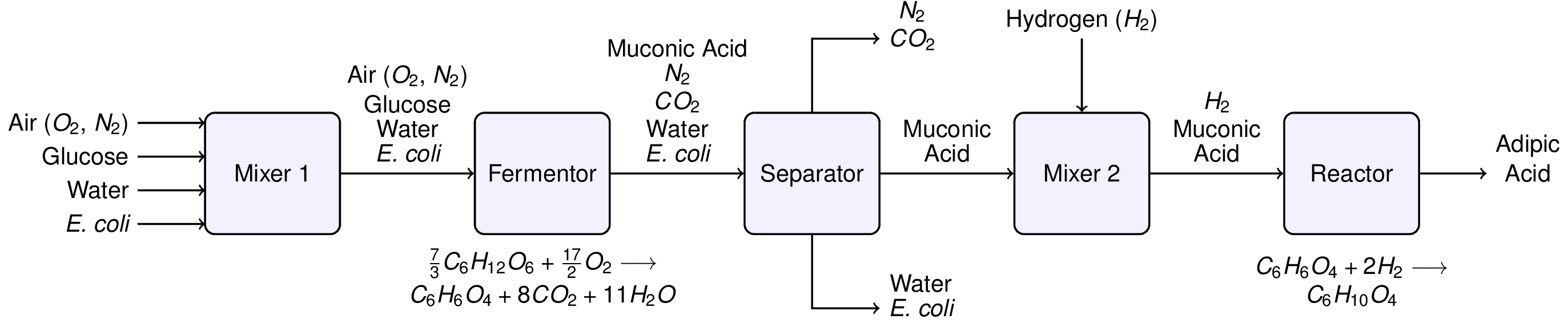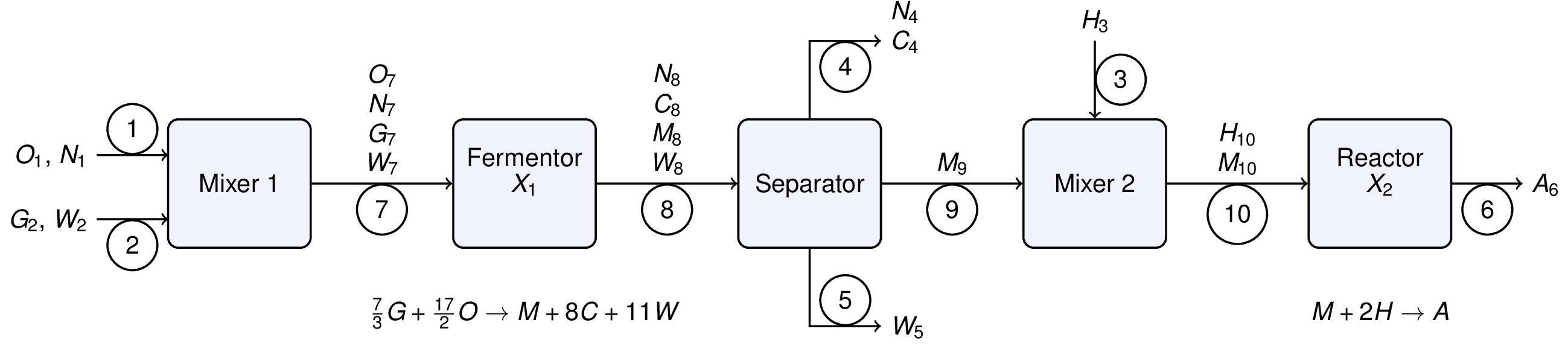This notebook contains course material from CBE20255 by Jeffrey Kantor (jeff at nd.edu); the content is available on Github. The text is released under the CC-BY-NC-ND-4.0 license, and code is released under the MIT license.

## Summary¶

This Jupyter notebook demonstrates the formulation and solution of material balances for a hypothetical adipic acid process described by Murphy (2005, Example 2.15) using the symbolic algebra package Sympy.

## Problem Statement¶

Adipic acid (C6H10O4) (also called hexanedioic acid) rarely occurs in nature, but approximately 2.5 billion kilograms are produced per year from petrochemical feedstocks primarily for the production of nylon.Recently there has been interest in producing adipic acid from renewable resources. For example, starting with glucose (C6H12O6), muconic acid (C6H6O4) is produced through fermentation with a genetically modified e. coli. via the reaction

73 C6H12O6 + 172 O2 ➝ C6H6O4 + 8 CO2 + 11 H2O

that is subsequently hydrogentated to form adipic acid

C6H6O4 + 2 H2 ➝ C6H10O4

Murphy (2005, Example 2.15) outlines a hypothetical flowsheet for this process:Neglecting the E. coli, solve for the flowrates necessary to produce 12,000 kg/hour of adipic acid assuming that glucose is available as a 10 mg/mL solution.

## Solution¶

### Process Variables¶

We begin by relabeling the process flowsheet with stream numbers, stream variables, and extents of reaction. There are chemical species are abbreviated as follows:

• C: carbon dioxide
• G: glucose
• H: hydrogen
• M: muconic acid
• N: nitrogen
• O: oxygen
• W: water

and where X1 and X2 denote the extents of reactions 1 and 2, respectively. The stream variables denote chemical flowrates in units of kg/hour. The extents of reaction will be in units of kg-mol/hour.In :
# Import the symbolic algebra package sympy
import sympy as sym

# Extents of reactions 1 and 2
sym.var('X1 X2')

# Stream variables
sym.var('O1 N1')           # Stream 1
sym.var('G2 W2')           # Stream 2
sym.var('H3')              # Stream 3
sym.var('N4 C4')           # Stream 4
sym.var('W5')              # Stream 5
sym.var('A6')              # Stream 6
sym.var('O7 N7 G7 W7')     # Stream 7
sym.var('N8 W8 C8 M8')     # Stream 8
sym.var('M9')              # Stream 9
sym.var('H10 M10')         # Stream 10

Out:
(H10, M10)

Because the flowsheet includes reactions, it will be necessary to include molecular weights in the mass balance expressions. For this purpose we gather the molecular weights of all species into a python dictionary indexed by the chemical species.

In :
MW = {
'A': 146.14,
'C': 44.01,
'G': 180.16,
'H': 2.02,
'M': 142.11,
'N': 14.01,
'O': 16.00,
'W': 18.02
}


### Specifications¶

In :
specs = [
sym.Eq(A6, 12000),
sym.Eq(N1/MW['N'], (0.79/0.21)*(O1/MW['O'])),
sym.Eq(G2, 0.01*W2)
]


### Material Balances¶

In :
mixer1 = [
sym.Eq(0, O1 - O7),
sym.Eq(0, N1 - N7),
sym.Eq(0, G2 - G7),
sym.Eq(0, W2 - W7)
]

reactor1 = [
sym.Eq(0,  O7 - MW['O']*(17/2)*X1),
sym.Eq(0,  N7 - N8),
sym.Eq(0,  G7 - MW['G']*(7/3)*X1),
sym.Eq(0, -C8 + MW['C']*8*X1),
sym.Eq(0, -M8 + MW['M']*X1),
sym.Eq(0,  W7 - W8 + MW['W']*11*X1)
]

separator = [
sym.Eq(0, N8 - N4),
sym.Eq(0, C8 - C4),
sym.Eq(0, M8 - M9),
sym.Eq(0, W8 - W5)
]

mixer2 = [
sym.Eq(0, M9 - M10),
sym.Eq(0, H3 - H10)
]

reactor2 = [
sym.Eq(0, H10 - MW['H']*2*X2),
sym.Eq(0, M10 - MW['M']*X2),
sym.Eq(0, -A6 + MW['A']*X2)
]

mbals = mixer1 + reactor1 + separator + mixer2 + reactor2

In :
specs + mbals

Out:
[Eq(A6, 12000),
Eq(0.0713775874375446*N1, 0.235119047619048*O1),
Eq(G2, 0.01*W2),
Eq(0, O1 - O7),
Eq(0, N1 - N7),
Eq(0, G2 - G7),
Eq(0, W2 - W7),
Eq(0, O7 - 136.0*X1),
Eq(0, N7 - N8),
Eq(0, G7 - 420.373333333333*X1),
Eq(0, -C8 + 352.08*X1),
Eq(0, -M8 + 142.11*X1),
Eq(0, W7 - W8 + 198.22*X1),
Eq(0, -N4 + N8),
Eq(0, -C4 + C8),
Eq(0, M8 - M9),
Eq(0, -W5 + W8),
Eq(0, -M10 + M9),
Eq(0, -H10 + H3),
Eq(0, H10 - 4.04*X2),
Eq(0, M10 - 142.11*X2),
Eq(0, -A6 + 146.14*X2)]

### Solution¶

In :
soln = sym.solve(mbals + specs)
soln

Out:
{H3: 331.736690844396,
C8: 28910.3599288354,
W2: 3451813.32968386,
W5: 3468089.77692623,
G7: 34518.1332968386,
N7: 36785.5285538330,
O7: 11167.3737511975,
W8: 3468089.77692623,
W7: 3451813.32968386,
N8: 36785.5285538330,
G2: 34518.1332968386,
N1: 36785.5285538330,
M9: 11669.0844395785,
C4: 28910.3599288354,
X2: 82.1130422882168,
M8: 11669.0844395785,
M10: 11669.0844395785,
A6: 12000.0000000000,
H10: 331.736690844396,
N4: 36785.5285538330,
O1: 11167.3737511975,
X1: 82.1130422882168}
In :
for key in soln.keys():
print("{:3s}    {:10.1f}".format(str(key), float(soln[key])))

H3          331.7
C8        28910.4
W2      3451813.3
W5      3468089.8
G7        34518.1
N7        36785.5
O7        11167.4
W8      3468089.8
W7      3451813.3
N8        36785.5
G2        34518.1
N1        36785.5
M9        11669.1
C4        28910.4
X2           82.1
M8        11669.1
M10       11669.1
A6        12000.0
H10         331.7
N4        36785.5
O1        11167.4
X1           82.1

In [ ]: